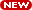## Math 128A Analysis of Numerical Methods - Spring 2004

Lectures: MWF 1-2 PM, 3 EVANS

Prof: M. Zworski, email zworski@math.berkeley.edu , WWW http://math.berkeley.edu/~zworski/

Office Hours: MW 11-12, F 2-3, and or by appointment, in 897 Evans.Office Hours during the exam week: W 2-4 PM, Th 2-4 PM.

Final Exam will be held on Saturday, May 22, in Evans 10, 12:30-3:30 PM.

Discussion section: TBA

GSI: Todor Milanov, milanov@math.berkeley.edu

GSI Office Hours: W, Th 11:15 AM - 12:15 PM, 745 Evans.

Course Web page: http://math.berkeley.edu/~zworski/128/

Course Control Number: 54915

Prerequisites: Math 53 and 54 or equivalent.

Required Text: R. L. Burden and J. D. Faires, Numerical Analysis, 7th edition, Brooks-Cole, 2001.

Syllabus: The course will cover basic theory and practical methods for solving the fundamental problems of computational science.

• Calculus review: Taylor series with remainder. Error analysis: roundoff and truncation errors in numerical approximations and computer arithmetic. Algorithms and convergence (Chapter 1).
• Nonlinear equations: bisection, fixed point, Newton and secant methods. Zeros of polynomials and Muller's method (Chapter 2).
• Polynomial interpolation. Lagrange vs. Hermite. Divided differences. Splines (Chapter 3).
• Numerical differentiation and integration: basic rules, extrapolation, adaptive and Gaussian strategies (Chapter 4).
• Initial value problems for ordinary differential equations. Runge-Kutta and Adams methods. Introduction to stiff problems (Chapter 5).
• Matrix computations: linear systems, LU factorization (Chapter 6).
• Approximation theory: least squares, Householder QR factorization, and orthogonal polynomials (Sections 7.1, 9.3 and 8.1-2).

Grading: Grades will be based on the best 10 problem sets (30%), midterm exams (30%), and final exam (40%). The problem sets will be due every Friday; late submissions cannot be accepted after solutions have been distributed.

Midterm Exam Dates: February 27 and April 2 (50 minute in class exams).